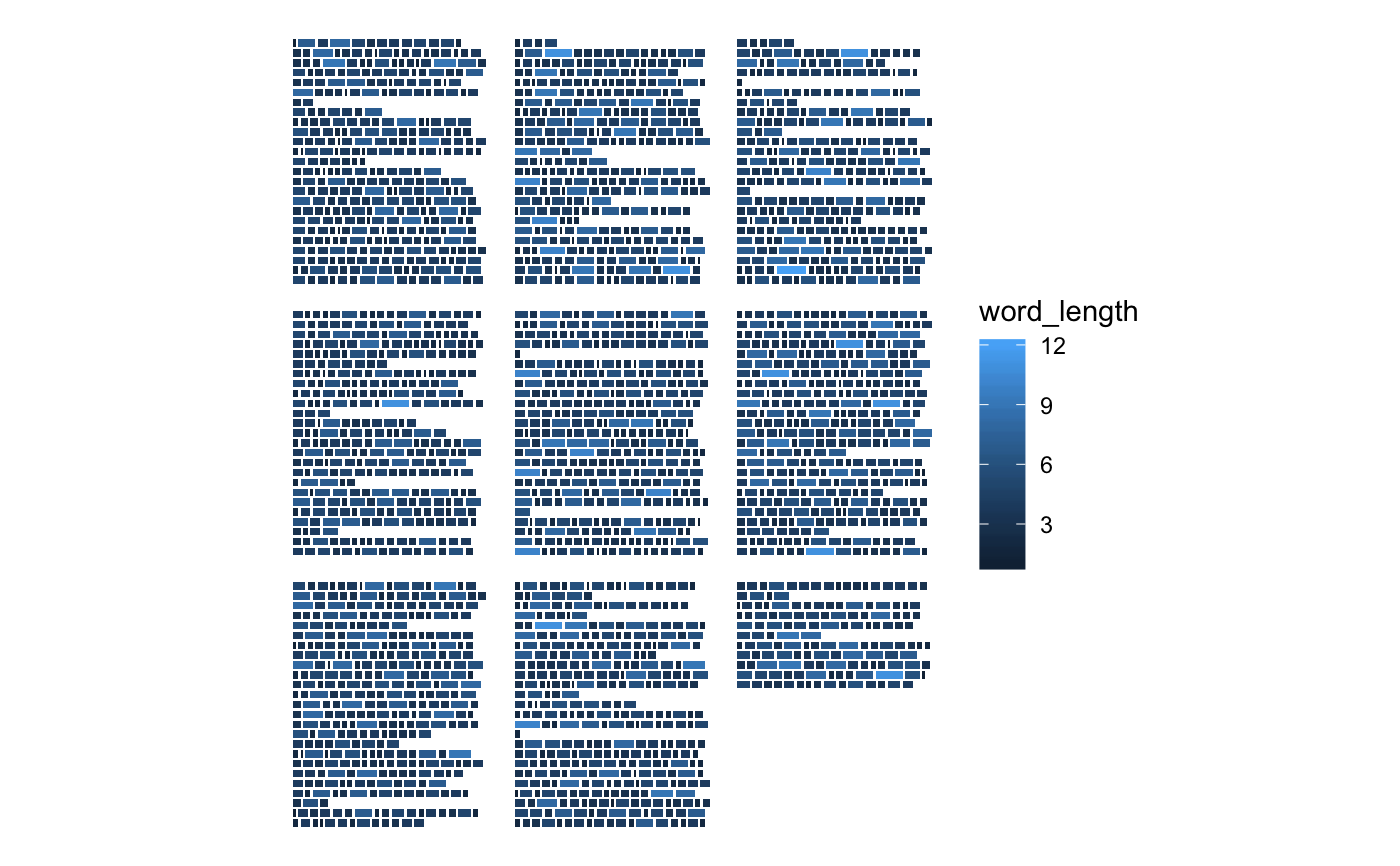Creates a visualization from the ggpage_build output

ggpage_plot(data, mapping = ggplot2::aes(), paper.show = FALSE,
paper.color = "grey90", paper.alpha = 1, paper.limits = 3,
page.number = character(1), page.number.x = 3, page.number.y = 3)

## Arguments

data data.frame. Expects output from ggpage_build with optional intermediate analysis. Default list of aesthetic mappings to use for plot to be handed to internal ggplot call. Shows the paper underneath the text. Color of the pages. Needs to be of length 1 or the same as the number of pages. Alpha of the pages. Needs to be of length 1 or the same as the number of pages. Numerical. Extends the edges of the paper in all directions. Position of the page number. Defaults to none. Distance the page number is pushed away from the text along the x-axis. Distance the page number is pushed away from the text along the y-axis.

## Value

A ggplot object with the given visualization.

## Examples

library(dplyr)
library(stringr)
library(ggplot2)
library(tidytext)
library(ggpage)
# build and plot
## data.frame with full lines
ggpage_build(tinderbox) %>%
ggpage_plot()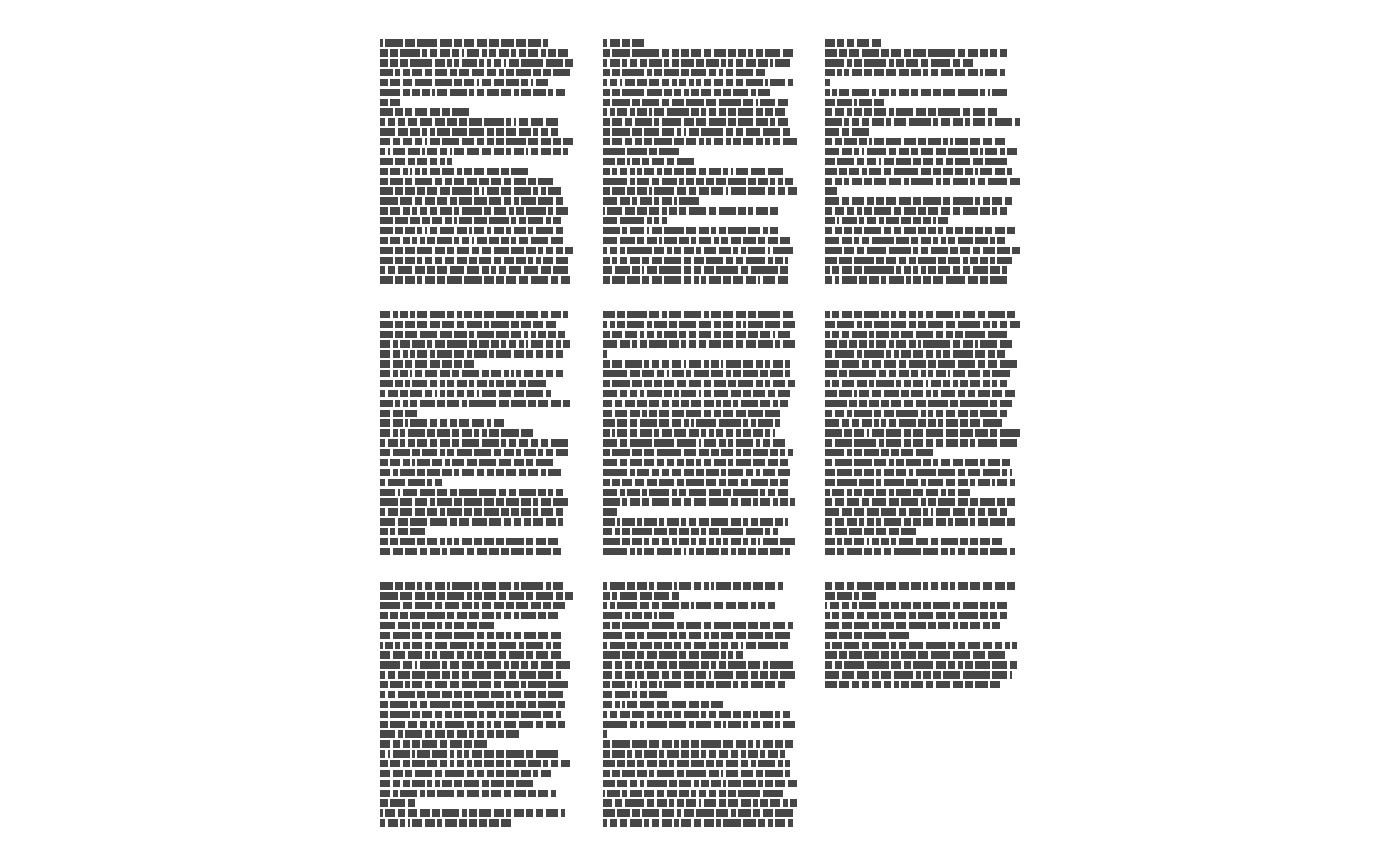## vector with full lines
ggpage_build(book = tinderbox %>%
pull(text)) %>%
ggpage_plot()## data.frame with single words
ggpage_build(tinderbox) %>%
unnest_tokens(text, word) %>%
ggpage_plot()## vector with single words
ggpage_build(tinderbox %>%
unnest_tokens(text, text) %>%
pull(text)) %>%
ggpage_plot()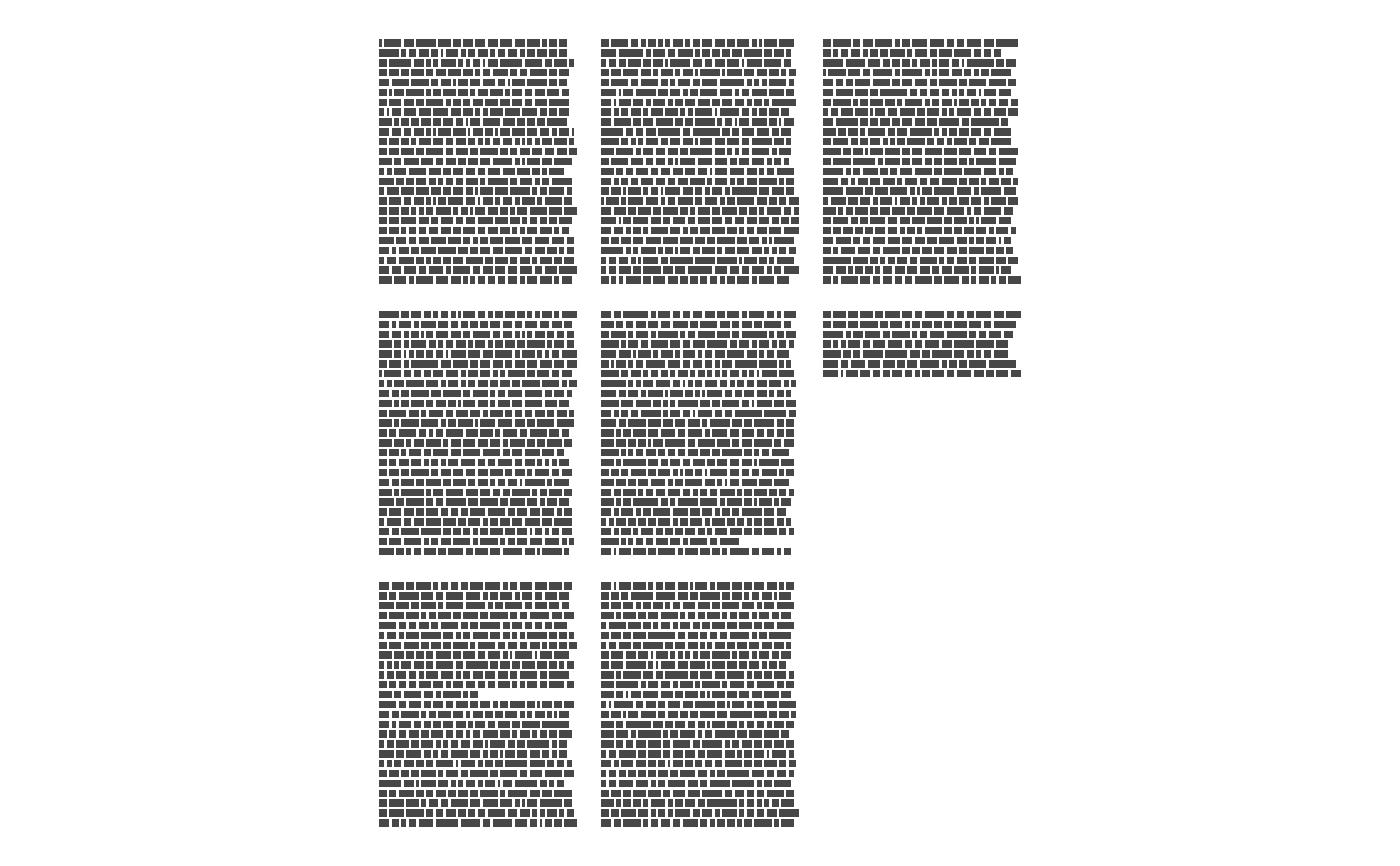# nrow and ncol
ggpage_build(tinderbox, nrow = 2) %>%
ggpage_plot()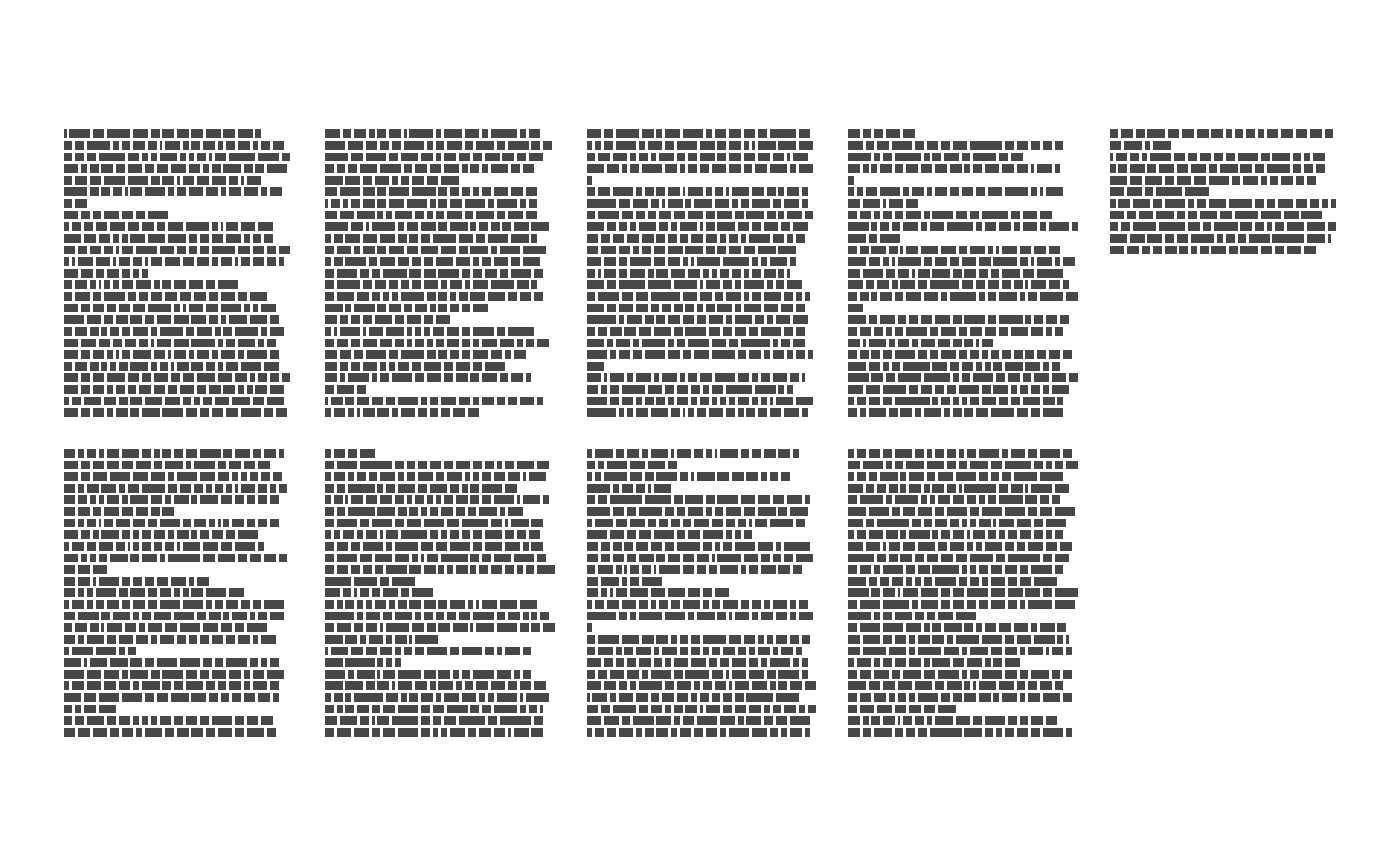ggpage_build(tinderbox, ncol = 2) %>%
ggpage_plot()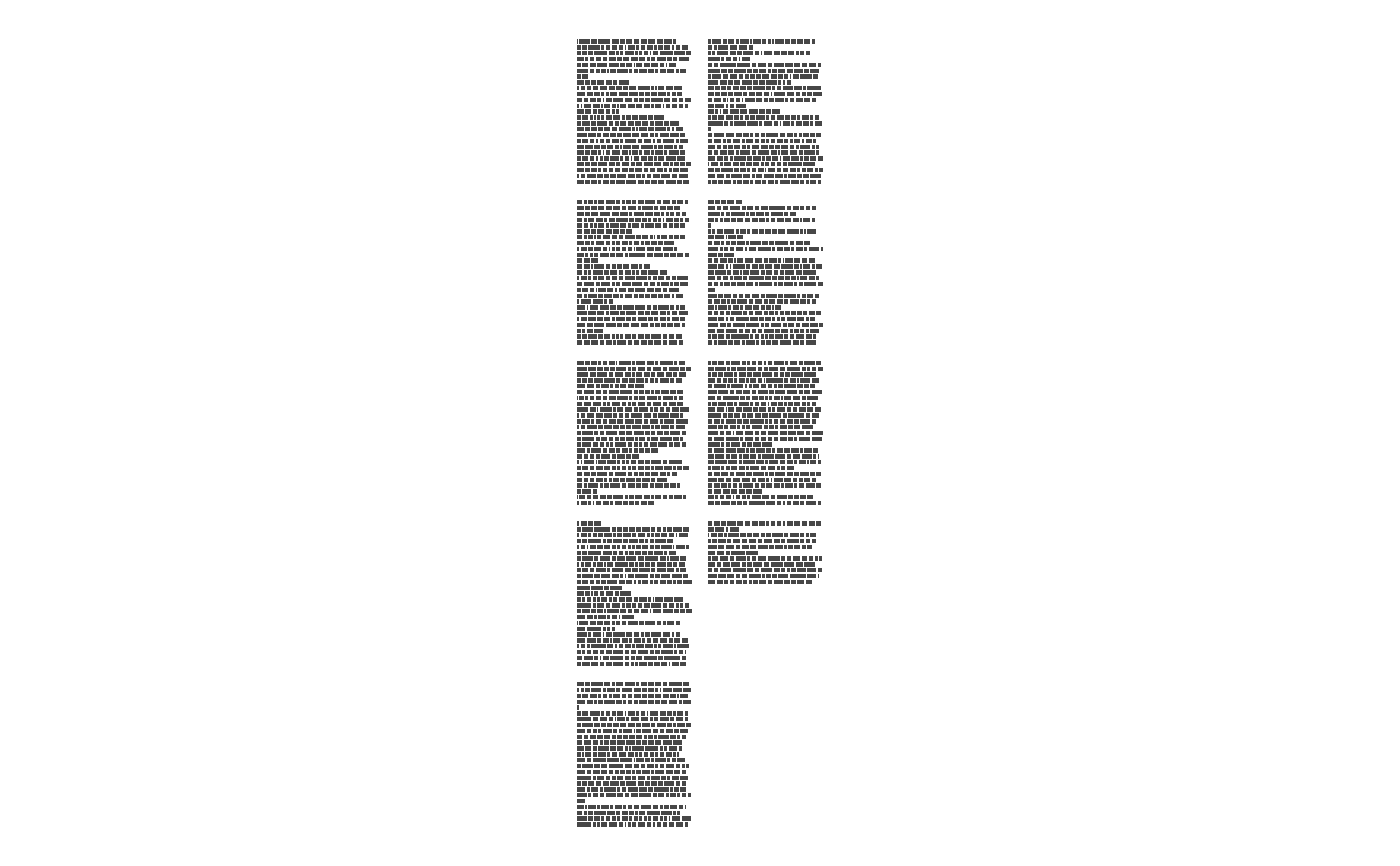# Include analysis within
ggpage_build(tinderbox) %>%
mutate(word_length = str_length(word)) %>%
ggpage_plot(aes(fill = word_length))